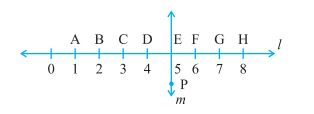Filter By

## All Questions

#### 4. Study the diagram. The lineis perpendicular to line(a) Is CE = EG?            (b) Does PE bisect CG?            (c) Identify any two line segments for which PE is the perpendicular bisector.            (d) Are these true?                    (i) AC > FG                    (ii) CD = GH                    (iii) BC < EH.(a) CE = 5 - 3 = 2 units

EG = 7 - 5 = 2 units

Therefore CE = EG.

(b) CE = EG therefore PE bisects CG.

(c) PE is the perpendicular bisector for line segments DF and BH

(d) (i) AC = 3 - 1 = 2 units

FG = 7 - 6 = 1 unit

Therefore AC > FG

True

(ii) CD = 4 - 3 = 1 unit

GH = 8 - 7 = 1 unit

Therefore CD = GH

True

(iii) BC = 3 - 2 = 1 unit

EH = 8 - 5 = 3 units

Therefore BC < EH

True

#### 3. There are two set-squares in your box. What are the measures of the angles that are formed at their corners? Do they have any angle measure that is common?

The angles of the two set quares are

(i) 90o, 60o and 30o

(ii) 90o, 45o and 45o

Yes they have the common angle measure 90o

## Crack CUET with india's "Best Teachers"

• HD Video Lectures
• Unlimited Mock Tests
• Faculty Support#### 2.  Letbe the perpendicular to the line segment. Letandintersect in the point A. What is the measure of?PQ and XY intersect at ATherefore#### 1.  Which of the following are models for perpendicular lines :            (a) The adjacent edges of a table top.            (b) The lines of a railway track.            (c) The line segments forming the letter ‘L’.            (d) The letter V.

(a) The adjacent edges of a table top are models for perpendicular lines.

(b) The lines of a railway track are not models for perpendicular lines as they are parallel to each other.

(c) The line segments forming the letter ‘L’ are models for perpendicular lines.

(d) The line segments forming the letter ‘V’ are models for perpendicular lines.

## Crack NEET with "AI Coach"

• HD Video Lectures
• Unlimited Mock Tests
• Faculty Support#### Q18         If u, v and w are functions of x, then show thatin two ways - first by repeated application of product rule, second by logarithmic differentiation.

It is given that u, v and w are the functions of x
LetNow, we differentiate using product rule w.r.t x
First, takeNow,-(i)
Now, again by the product rulePut this in equation (i)
we get,Hence, by product rule we proved it

Now, by taking the log
Again takeNow, take log on both sidesNow, differentiate w.r.t. x
we get,Hence, we proved it by taking the log

#### Q17 (3)   Differentiatein three ways mentioned below:               (iii)  by logarithmic differentiation.                  Do they all give the same answer?

Given function isNow, take log on both the sidesNow, differentiate w.r.t. x
we get,Therefore, the answer isAnd yes they all give the same answer

## Crack JEE Main with "AI Coach"

• HD Video Lectures
• Unlimited Mock Tests
• Faculty Support#### Q17 (2)   Differentiatein three ways mentioned below:                 (ii) by expanding the product to obtain a single polynomial.

Given function isMultiply both to  obtain a single higher degree polynomialNow, differentiate w.r.t. x
we get,Therefore, the answer is#### Q17 (1)   Differentiatein three ways mentioned below:                 (i) by using product rule

Given function  isNow, we need to differentiate using the product ruleTherefore, the answer is## Crack CUET with india's "Best Teachers"

• HD Video Lectures
• Unlimited Mock Tests
• Faculty Support#### Q16  Find the derivative of the function given byand hence find            f ' (1)

Given function isTake log on both sidesNOW, differentiate w.r.t. xTherefore,Now, the vale ofis#### Q15  Find dy/dx of the functions given in Exercises 12 to 15.Given function isNow, take  take log on both the sidesNow, differentiate w.r.t  xBy taking similar terms on same side
We get,Therefore, the answer is